## 知识图谱：最短路径算法Bellman-Ford

• 张丹, 分析师/程序员/Quant: R,Java,Nodejs
• blog: http://blog.fens.me
• email: bsspirit@gmail.com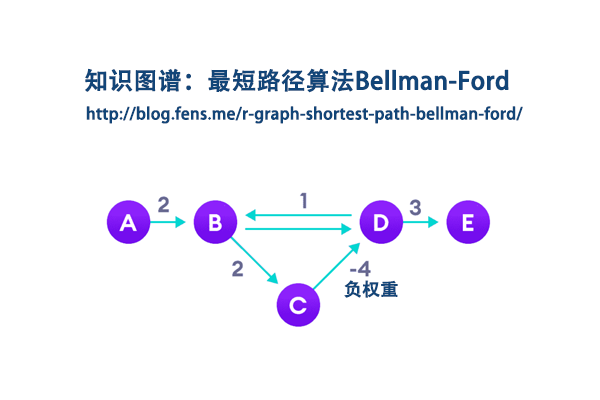1. Bellman-Ford算法介绍
2. R语言调用Bellman-Ford算法
3. 自己手写Bellman-Ford算法
4. 算一个复杂点的网络关系图

## 1. Bellman-Ford算法介绍

Bellman Ford算法最初于1955年被Alfonso Shimbel提出，但最终基于1958和1956年发表论文的 Richard Bellman和Lester Ford, Jr.二人命名。Edward F. Moore于1957年也提出了该算法，因此该算法有时也称作是Bellman Ford Moore算法。

Bellman-Ford算法与Dijkstra算法一样，解决的是单源最短路径问题。两者不同之处在于，Dijkstra只适用于无负权边的图，而Bellman-Ford算法无此限制：无论是否有向，无论是否有负权边，只要图中没有负权环，则该算法可以正确地给出起点到其余各点的最短路径，否则报告负权环的存在。

Bellman-Ford 算法采用动态规划进行设计，实现的时间复杂度为 O(V*E)，其中 V 为顶点数量，E 为边的数量。每次松弛操作实际上是对相邻节点的访问(相当于广度优先搜索)，第n次松弛操作保证了所有深度为n的路径最短。由于图的最短路径最长不会经过超过|V| – 1条边，所以可知贝尔曼-福特算法所得为最短路径，也可只时间复杂度为O(VE)。

Bellman-Ford 算法描述：

• 创建源顶点 v 到图中所有顶点的距离的集合 distSet，为图中的所有顶点指定一个距离值，初始均为 Infinite，源顶点距离为 0；
• 计算最短路径，执行 V – 1 次遍历；
• 对于图中的每条边：如果起点 u 的距离 d 加上边的权值 w 小于终点 v 的距离 d，则更新终点 v 的距离值 d；
• 检测图中是否有负权边形成了环，遍历图中的所有边，计算 u 至 v 的距离，如果对于 v 存在更小的距离，则说明存在环；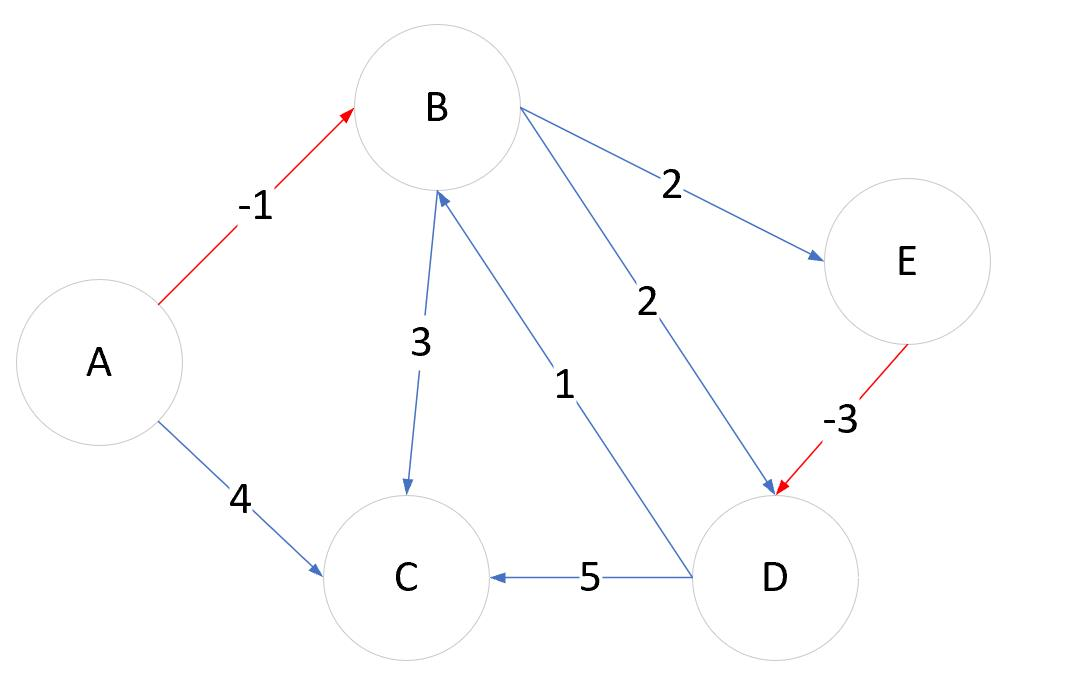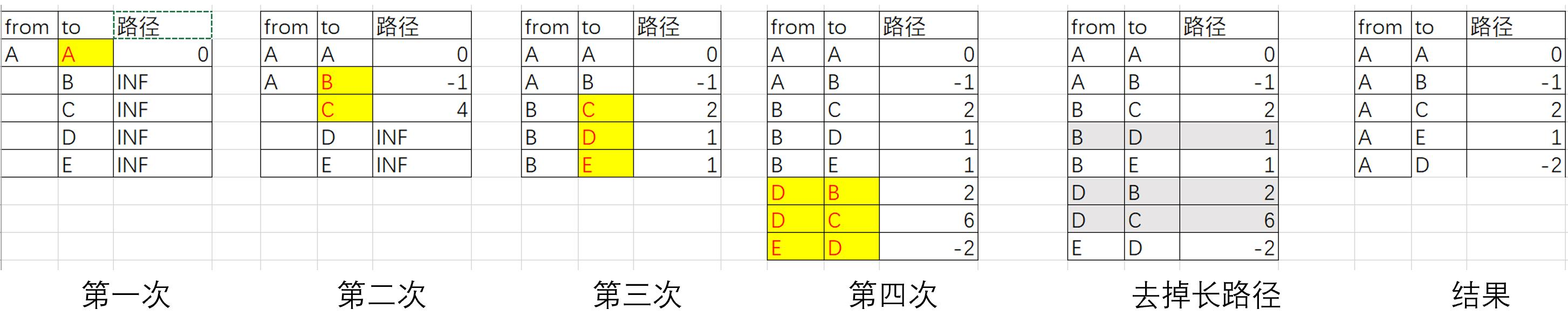## 2. R语言调用Bellman-Ford算法

``````# 加载igraph库
> library(igraph)

# 新建图数据
> df<-data.frame(
+   from=c("A","A","B","B","B","D","D","E"),
+   to=c("B","C","C","D","E","B","C","D"),
+   weight=c(-1,4,3,2,2,1,5,-3)
+ )

# 用igraph画图
> a<-graph_from_data_frame(df, directed = TRUE)
> E(a)\$arrow.size<-1
> E(a)\$arrow.width<-0.2
> E(a)\$label<-df\$weight

# layout需要设置一下，不然图的展示会很不好看
> plot(a,layout=layout.gem)
``````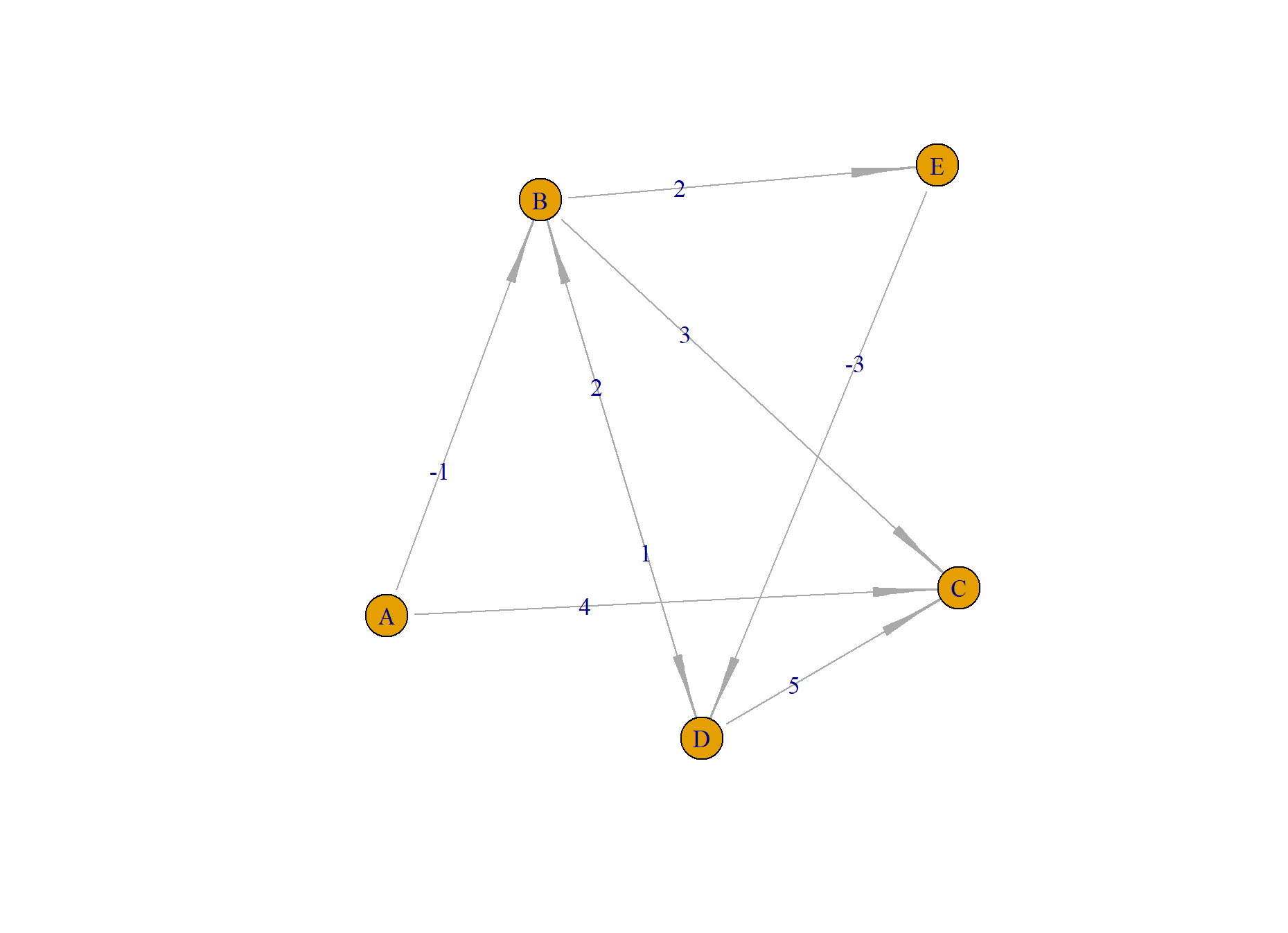layout需要设置一下，不然图的展示会节点叠加在一起，就看不出来图的样子了，我这里选择layout.gem类型。

``````# 使用bellman-ford算法
> shortest.paths(a,"A", algorithm = "bellman-ford")
Error in shortest.paths(a, "A", algorithm = "bellman-ford") :
At core/paths/bellman_ford.c:157 : cannot run Bellman-Ford algorithm, Negative loop detected while calculating shortest paths
``````

## 3. 自已手写Bellman-Ford算法

``````# bellman-ford 最短距离算法
# @param df数据集
# @param start开始节点
bellman_ford<-function(df,start="A"){

# 初始化结果数据集
rs<-data.frame(from=start,to=start,weight=0)

# 初始化结束节点
finishnode<-c()

# 初始化开始节点
nodes<-rs

# 当开始节点数量<结束节点数量时，继续循环计算
while(length(finishnode)<length(rs\$to)){

# 过滤已计算过的结束节点
if(length(finishnode)>0){
nodes<-rs[-which(rs\$to %in% finishnode),]
}

# 对原始数据进行循环，遍历每一行
for(i in 1:nrow(df)){
row<-df[i,];row

# 对结束节点进行循环，遍历每一个结束节点
for(j in nodes\$to){
if(row\$from==j){
# 把之前结束节点的权重叠加本次计算中
row\$weight<-row\$weight+rs\$weight[which(rs\$to==j)]
# 把结束节点加入到结果数据集
rs<-rbind(rs,row)
}
}
}

# 保留最短的唯一结束节点
rs2<-rs[order(rs\$weight),]
n<-which(duplicated(rs2\$to))
if(length(n)<0){
rs2<-rs2[-n,]
rs<-rs2[order(as.numeric(row.names(rs2))),]
}

# 更新已经完成的结束节点
finishnode<-c(finishnode,nodes\$to)
}
return(rs)
}
``````

``````> rs<-bellman_ford(df,"A")
> rs
from to weight
1    A  A      0
2    A  B     -1
3    B  C      2
5    B  E      1
8    E  D     -2
``````

``````# 计算2点间的最短路径
# @param rs 结果数据集
# @param start 开始节点
# @param end 结束节点
get_shortest_path<-function(rs,start,end){

# 初始化节点
q<-c(start)
idx<-which(rs\$from==start)

# 判断有几个分支
if(length(idx)>0){
for(i in idx){
# 递归计算
q2<-get_shortest_path(rs,rs\$to[i],end)
if(tail(q2,1)==end){
q<-c(q,q2)
}
}
}

# 查看队列状态
# print(q)

# 当找到结束节点，程序停止
if(tail(q,1)==end){
return(q)
}
return(q)
}

# 计算从开始节点到结束节点的路径
> path<-get_shortest_path(rs[-1,],"A","D")
> path
 "A" "B" "E" "D"
``````

``````# 把路径合并成data.frame
> path2<-data.frame(from=path[-length(path)],to=path[-1])
# 找到最短路径的索引
> idx<-which(paste0(df\$from,"-",df\$to) %in% paste0(path2\$from,"-",path2\$to))

# 涂色
> df\$color<-"gray"
> df\$color[idx]<-"red"
> E(a)\$color<-df\$color
> plot(a,layout=layout.gem)
``````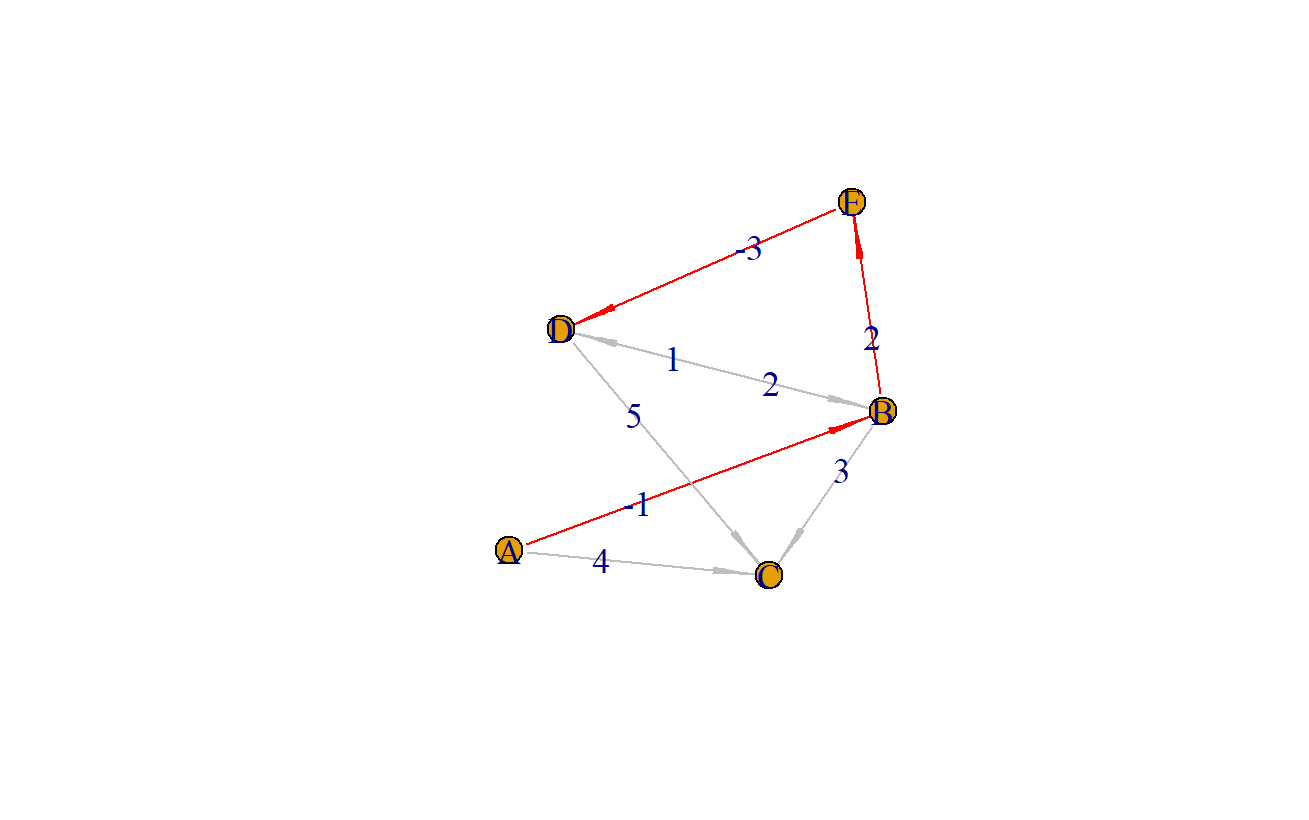## 4. 算一个复杂点的网路关系图

``````> df2<-data.frame(
+   from=c("A","B","C","D","C","F","B","B","F","G","H","F","E"),
+   to=  c("B","C","D","C","E","E","F","G","G","H","I","I","J"),
+   weight=c(5,20,10,-5,75,25,30,60,5,-50,-10,50,100)
+ )
``````

``````> a2<-graph_from_data_frame(df2, directed = TRUE)
> E(a2)\$arrow.size<-1
> E(a2)\$arrow.width<-0.2
> E(a2)\$label<-df2\$weight
> plot(a2,layout=layout.gem)
``````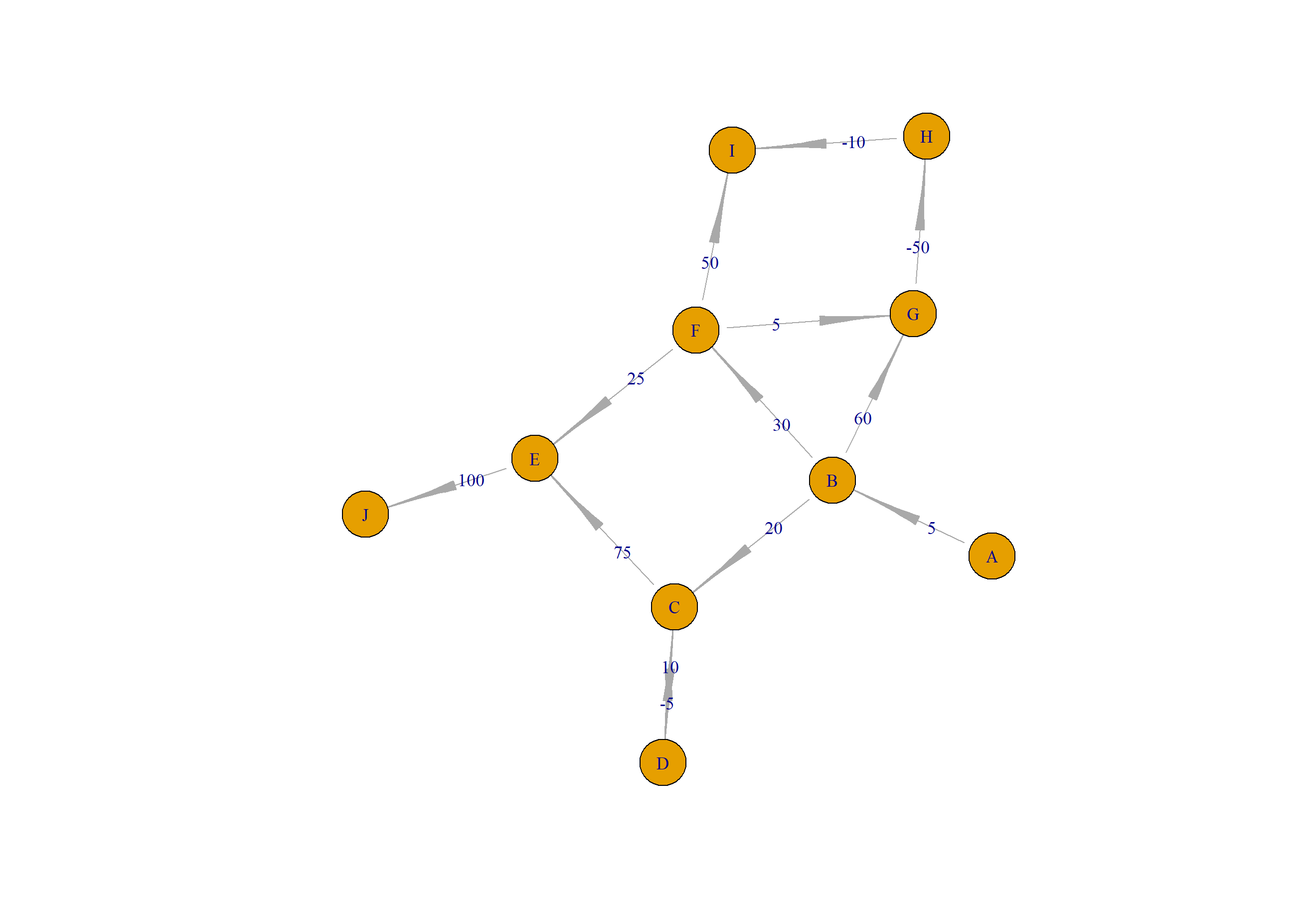``````> rs<-bellman_ford(df2,"A")
> rs
from to weight
1     A  A      0
2     A  B      5
3     C  D     35
6     F  E     60
7     B  F     35
9     F  G     40
10    G  H    -10
11    H  I    -20
13    E  J    160
21    B  C     25
``````

``````# 计算 A 到 D的最短路径
> path<-get_shortest_path(rs[-1,],"A","D")
> path
 "A" "B" "C" "D"

# 画图
> path2<-data.frame(from=path[-length(path)],to=path[-1])
> idx<-which(paste0(df2\$from,"-",df2\$to) %in% paste0(path2\$from,"-",path2\$to))
> df2\$color<-"gray"
> df2\$color[idx]<-"red"
> E(a2)\$arrow.size<-1
> E(a2)\$arrow.width<-0.2
> E(a2)\$color<-df2\$color
> plot(a2,layout=layout.gem)
``````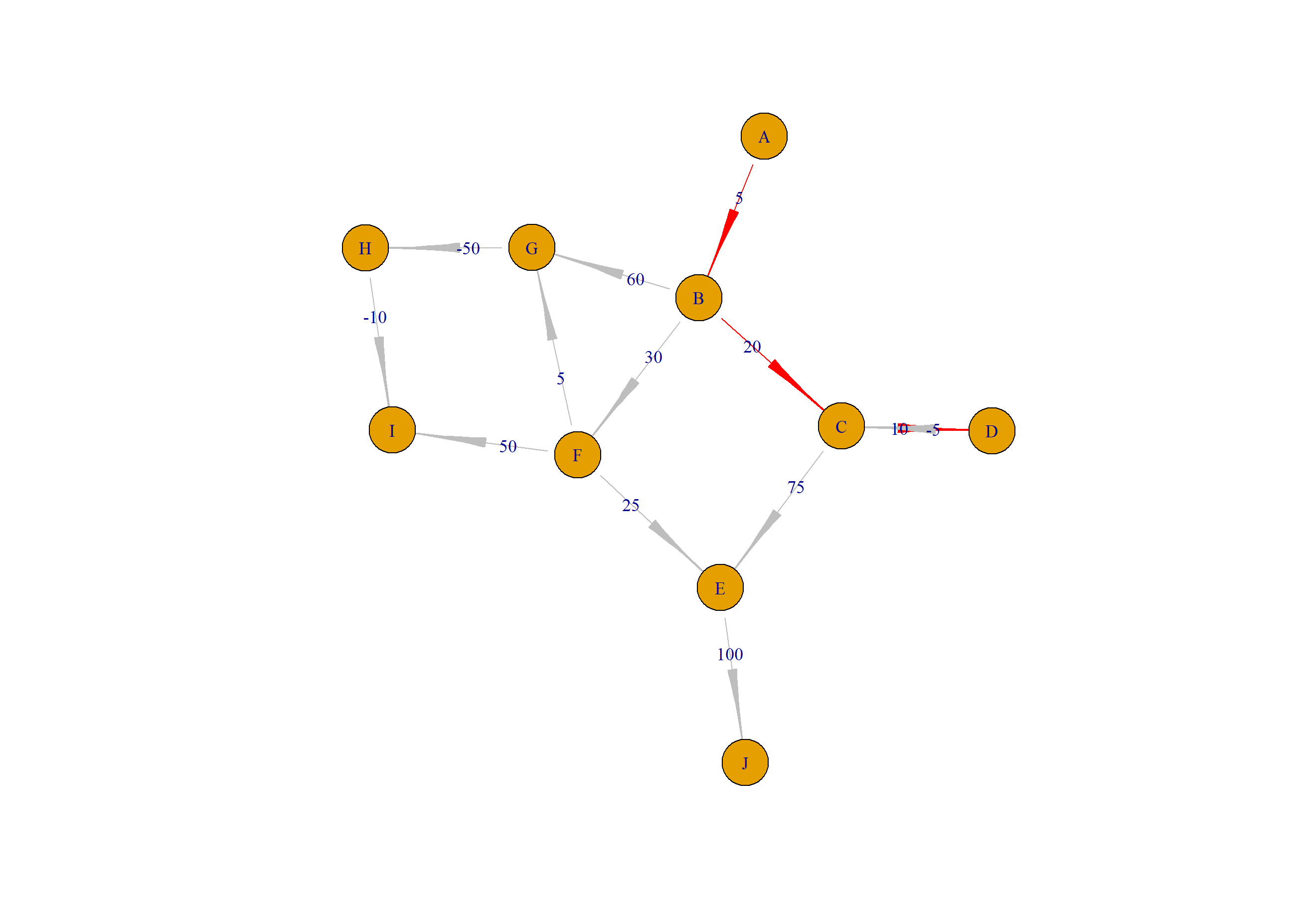``````# 计算 A 到 I的最短路径
> path<-get_shortest_path(rs[-1,],"A","I")
> path
 "A" "B" "F" "G" "H" "I"

# 画图
> path2<-data.frame(from=path[-length(path)],to=path[-1])
> idx<-which(paste0(df2\$from,"-",df2\$to) %in% paste0(path2\$from,"-",path2\$to))
> df2\$color<-"gray"
> df2\$color[idx]<-"red"
> E(a2)\$arrow.size<-1
> E(a2)\$arrow.width<-0.2
> E(a2)\$color<-df2\$color
> plot(a2,layout=layout.gem)
``````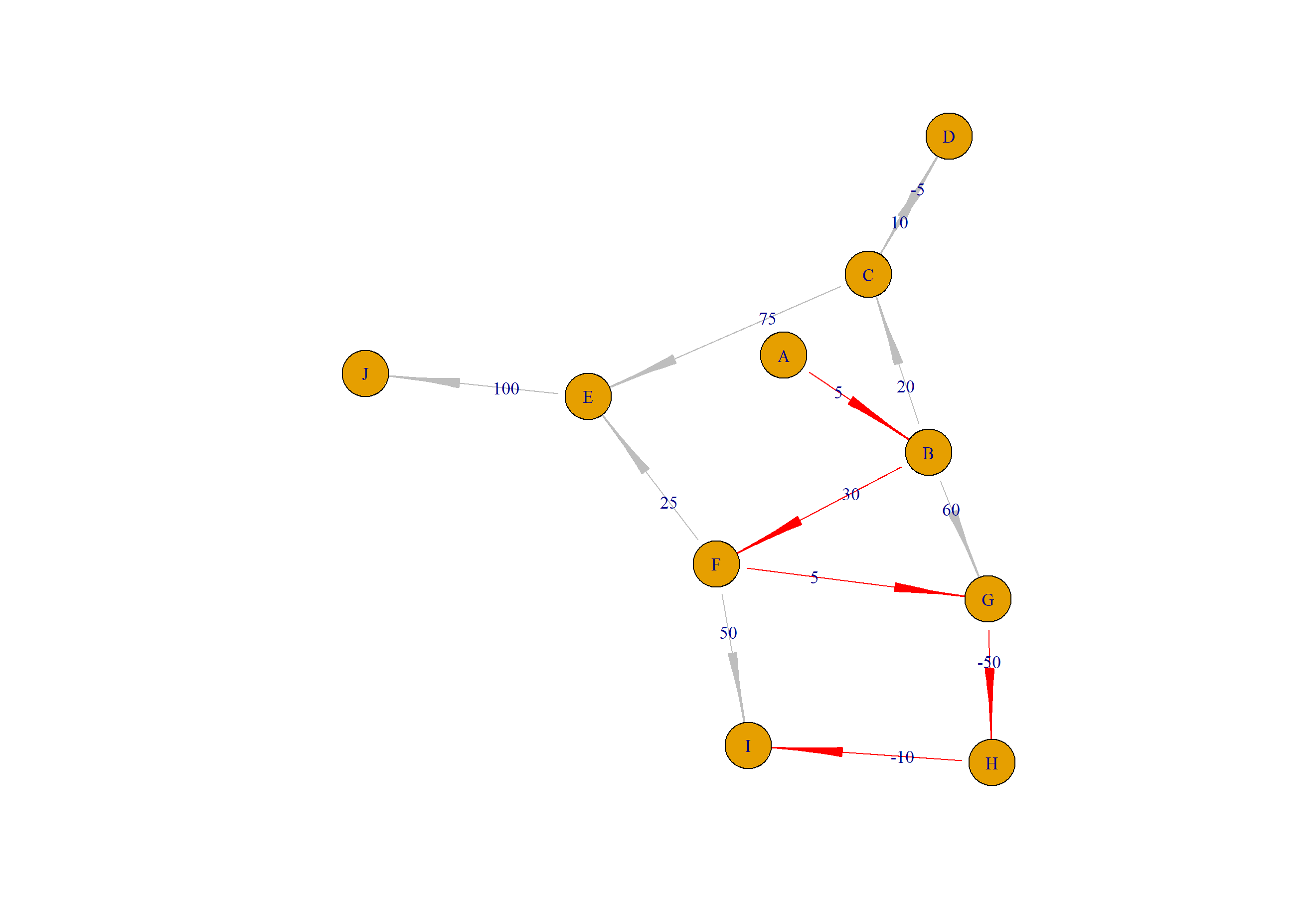http://blog.fens.me/r-graph-shortest-path-bellman-ford/This entry was posted in R语言实践, 程序算法

Article Rating
Subscribe
Notify ofThis site uses Akismet to reduce spam. Learn how your comment data is processed.

1 Comment
Inline Feedbacks